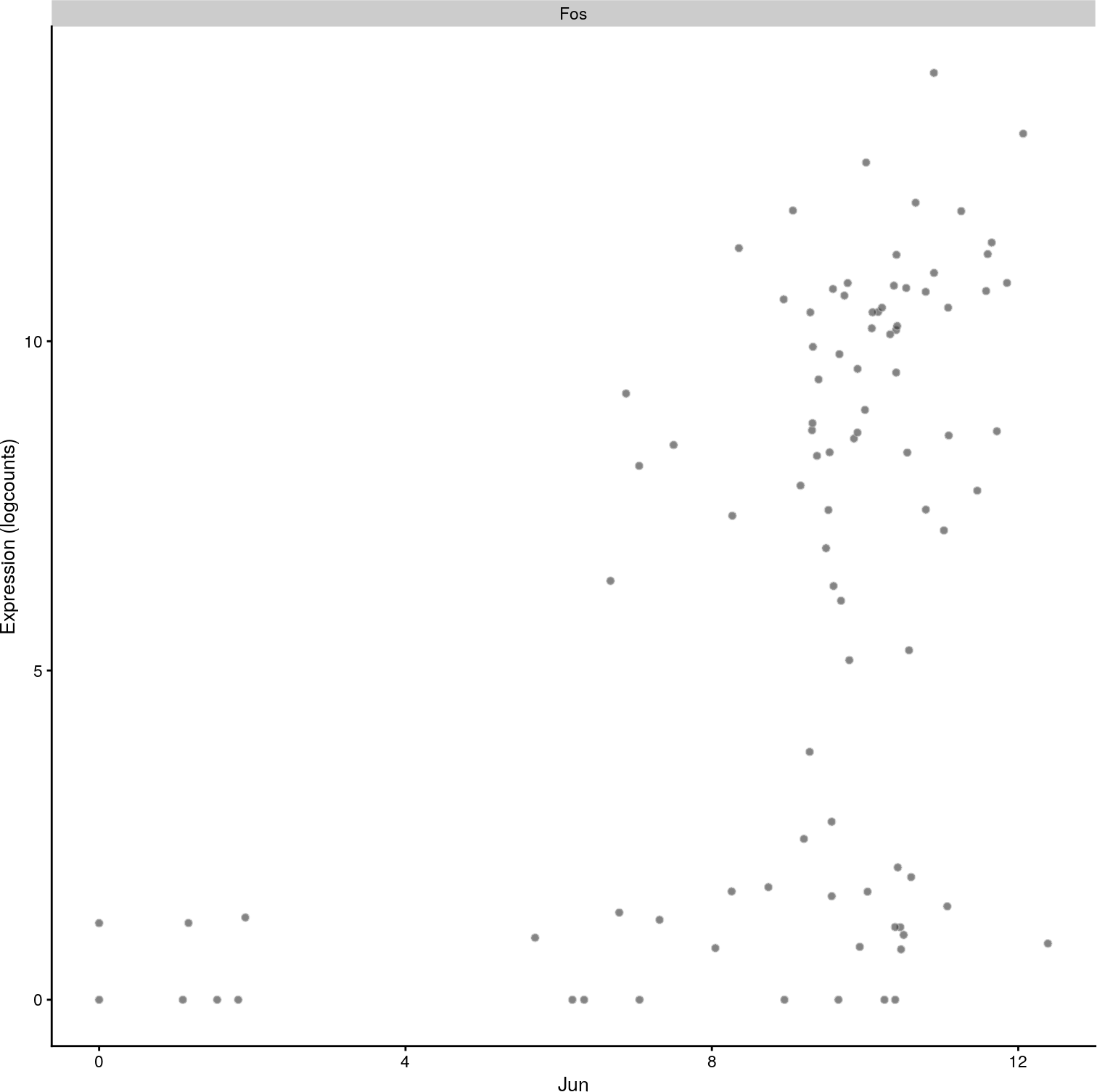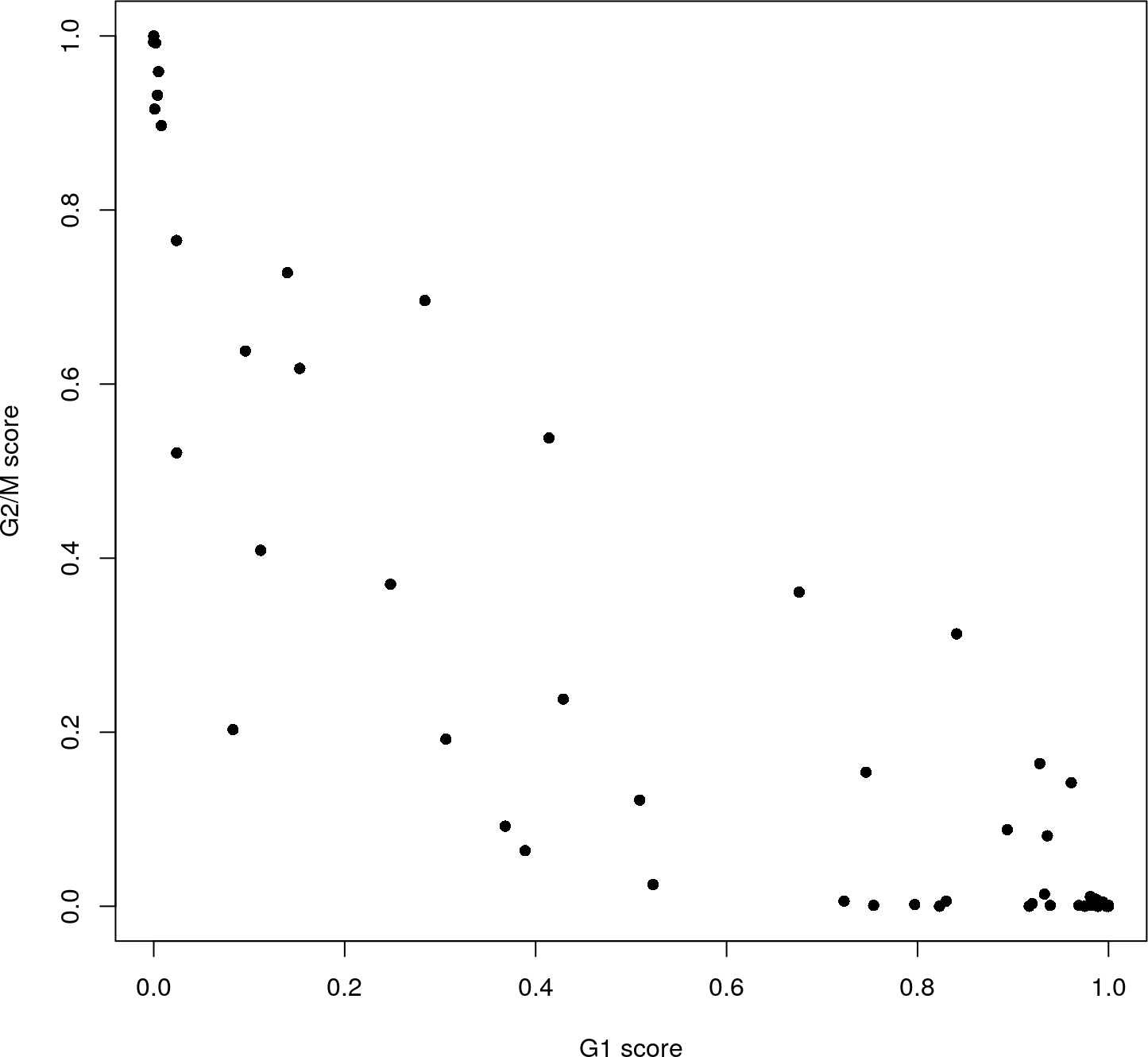# 1 Overview

Here, we describe a few additional analyses that can be performed with single-cell RNA sequencing data. This includes detection of significant correlations between genes and regressing out the effect of cell cycle from the gene expression matrix.

# 2 Identifying correlated gene pairs with Spearman’s rho

scRNA-seq data is commonly used to identify correlations between the expression profiles of different genes. This is quantified by computing Spearman’s rho, which accommodates non-linear relationships in the expression values. Non-zero correlations between pairs of genes provide evidence for their co-regulation. However, the noise in the data requires some statistical analysis to determine whether a correlation is significantly non-zero.

To demonstrate, we use the `correlatePairs` function to identify significant correlations between the various histocompatability antigens in the haematopoietic stem cell (HSC) Smart-seq2 dataset (Wilson et al. 2015). Counts were obtained from NCBI GEO as a supplementary file using the accession number GSE61533, and are used to generate a `SingleCellExperiment` as shown below.

``````library(BiocFileCache)
wilson.fname <- bfcrpath(bfc, file.path("ftp://ftp.ncbi.nlm.nih.gov/geo/series",
"GSE61nnn/GSE61533/suppl/GSE61533_HTSEQ_count_results.xls.gz"))
library(R.utils)
wilson.name2 <- "GSE61533_HTSEQ_count_results.xls"
gunzip(wilson.fname, destname=wilson.name2, remove=FALSE, overwrite=TRUE)

gene.names <- all.counts\$ID
all.counts <- as.matrix(all.counts[,-1])
rownames(all.counts) <- gene.names

library(SingleCellExperiment)
sce.hsc <- SingleCellExperiment(list(counts=all.counts))
is.spike <- grepl("^ERCC", rownames(sce.hsc))
sce.hsc <- splitAltExps(sce.hsc, ifelse(is.spike, "ERCC", "gene"))

library(scater)
spike.drop <- quickPerCellQC(colData(sce.hsc))

library(scran)
sce.hsc <- computeSumFactors(sce.hsc)
sce.hsc <- logNormCounts(sce.hsc)``````

The significance of each correlation is determined using a permutation test. For each pair of genes, the null hypothesis is that the expression profiles of two genes are independent. Shuffling the profiles and recalculating the correlation yields a null distribution that is used to obtain a p-value for each observed correlation value (Phipson and Smyth 2010).

``````set.seed(100)
var.cor <- correlatePairs(sce.hsc, subset.row=grep("^H2-", rownames(sce.hsc)))
``````## DataFrame with 6 rows and 6 columns
##         gene1       gene2       rho     p.value        FDR   limited
##   <character> <character> <numeric>   <numeric>  <numeric> <logical>
## 1       H2-Aa      H2-Ab1  0.529774 2.00000e-06 0.00189200      TRUE
## 2       H2-D1       H2-K1  0.436215 7.99999e-06 0.00378400     FALSE
## 3      H2-Ab1      H2-Eb1  0.422085 2.20000e-05 0.00693733     FALSE
## 4       H2-Aa      H2-Eb1  0.405762 4.40000e-05 0.01040599     FALSE
## 5       H2-Q6       H2-Q7  0.337576 9.43999e-04 0.17860462     FALSE
## 6       H2-K1       H2-K2  0.316598 2.05000e-03 0.32321634     FALSE``````

Correction for multiple testing across many gene pairs is performed by controlling the FDR at 5%.

``````sig.cor <- var.cor\$FDR <= 0.05
summary(sig.cor)``````
``````##    Mode   FALSE    TRUE
## logical     942       4``````

We can also compute correlations between specific pairs of genes, or between all pairs between two distinct sets of genes. The example below computes the correlation between Fos and Jun, which dimerize to form the AP-1 transcription factor (Angel and Karin 1991).

``correlatePairs(sce.hsc, subset.row=cbind("Fos", "Jun"))``
``````## DataFrame with 1 row and 6 columns
##         gene1       gene2       rho     p.value         FDR   limited
##   <character> <character> <numeric>   <numeric>   <numeric> <logical>
## 1         Fos         Jun  0.449599 7.99999e-06 7.99999e-06     FALSE``````

Examination of the expression profiles in Figure 1 confirms the presence of a modest correlation between these two genes.

``````library(scater)
plotExpression(sce.hsc, features="Fos", x="Jun")``````Figure 1: Expression of Fos plotted against the expression of Jun for all cells in the HSC dataset.

The use of `correlatePairs` is primarily intended to identify correlated gene pairs for validation studies. Obviously, non-zero correlations do not provide evidence for a direct regulatory interaction, let alone specify causality. To construct regulatory networks involving many genes, we suggest using dedicated packages such as WCGNA.

• We suggest only computing correlations between a subset of genes of interest, known either a priori or empirically defined, e.g., as HVGs. Computing correlations across all genes will take too long; unnecessarily increase the severity of the multiple testing correction; and may prioritize strong but uninteresting correlations, e.g., between tightly co-regulated house-keeping genes.
• The `correlateGenes()` function can be used on the output of `correlatePairs()` to return gene-centric output. This calculates a combined p-value (Simes 1986) for each gene that indicates whether it is significantly correlated to any other gene. From a statistical perspective, this is a more natural approach to correcting for multiple testing when genes, rather than pairs of genes, are of interest.
• The `Limited` field indicates whether the p-value was lower-bounded by the number of permutations. If this is `TRUE` for any non-significant gene at the chosen FDR threshold, consider increasing the number of permutations to improve power.

# 3 Comments on filtering by abundance

Low-abundance genes are problematic as zero or near-zero counts do not contain much information for reliable statistical inference. In applications involving hypothesis testing, these genes typically do not provide enough evidence to reject the null hypothesis yet they still increase the severity of the multiple testing correction. The discreteness of the counts may also interfere with statistical procedures, e.g., by compromising the accuracy of continuous approximations. Thus, low-abundance genes are often removed in many RNA-seq analysis pipelines before the application of downstream methods.

The “optimal” choice of filtering strategy depends on the downstream application. A more aggressive filter is usually required to remove discreteness and to avoid zeroes, e.g., for normalization purposes. By comparison, the filter statistic for hypothesis testing is mainly required to be independent of the test statistic under the null hypothesis (Bourgon, Gentleman, and Huber 2010). Given these differences in priorities, we (or the relevant function) will filter at each step as appropriate, rather than applying a single filter for the entire analysis. For example, `computeSumFactors()` will apply a somewhat stringent filter based on the average count, while `fitTrendVar()` will apply a relatively relaxed filter based on the average log-expression. Other applications will not do any abundance-based filtering at all (e.g., `denoisePCA()`) to preserve biological signal from lowly expressed genes.

Nonetheless, if global filtering is desired, it is simple to achieve by simply subsetting the `SingleCellExperiment` object. The example below demonstrates how we could remove genes with average counts less than 1 in the HSC dataset. The number of `TRUE` values in `demo.keep` corresponds to the number of retained rows/genes after filtering.

``````ave.counts <- calculateAverage(sce.hsc)
demo.keep <- ave.counts >= 1
filtered.sce.hsc <- sce.hsc[demo.keep,]
summary(demo.keep)``````
``````##    Mode   FALSE    TRUE
## logical   24377   14029``````

# 4 Blocking on the cell cycle phase

Cell cycle phase is usually uninteresting in studies focusing on other aspects of biology. However, the effects of cell cycle on the expression profile can mask other effects and interfere with the interpretation of the results. This cannot be avoided by simply removing cell cycle marker genes, as the cell cycle can affect a substantial number of other transcripts (Buettner et al. 2015). Rather, more sophisticated strategies are required, one of which is demonstrated below using data from a study of T Helper 2 (TH2) cells (Mahata et al. 2014).

``````library(BiocFileCache)
bfc <- BiocFileCache("raw_data", ask = FALSE)
mahata.fname <- bfcrpath(bfc,
"http://www.nature.com/nbt/journal/v33/n2/extref/nbt.3102-S7.xlsx")``````

Buettner et al. (2015) have already applied quality control and normalized the data, so we can use them directly as log-expression values (accessible as Supplementary Data 1 of https://dx.doi.org/10.1038/nbt.3102).

``````library(readxl)
rownames(incoming) <- incoming[,1]
incoming <- incoming[,-1]
incoming <- incoming[,!duplicated(colnames(incoming))] # Remove duplicated genes.
sce.th2 <- SingleCellExperiment(list(logcounts=t(incoming)))``````

We empirically identify the cell cycle phase using the pair-based classifier in `cyclone`. The majority of cells in Figure 2 seem to lie in G1 phase, with small numbers of cells in the other phases.

``````library(org.Mm.eg.db)
ensembl <- mapIds(org.Mm.eg.db, keys=rownames(sce.th2), keytype="SYMBOL", column="ENSEMBL")

set.seed(100)
package="scran"))
assignments <- cyclone(sce.th2, mm.pairs, gene.names=ensembl, assay.type="logcounts")

plot(assignments\$score\$G1, assignments\$score\$G2M,
xlab="G1 score", ylab="G2/M score", pch=16)``````Figure 2: Cell cycle phase scores from applying the pair-based classifier on the TH2 dataset, where each point represents a cell.

We can block directly on the phase scores in downstream analyses. This is more graduated than using a strict assignment of each cell to a specific phase, as the magnitude of the score considers the uncertainty of the assignment. The phase covariates in the design matrix will absorb any phase-related effects on expression such that they will not affect estimation of the effects of other experimental factors. Users should also ensure that the phase score is not confounded with other factors of interest. For example, model fitting is not possible if all cells in one experimental condition are in one phase, and all cells in another condition are in a different phase.

``````design <- model.matrix(~ G1 + G2M, assignments\$score)
dec.block <- modelGeneVar(sce.th2, design=design)

library(limma)
sce.th2.block <- sce.th2
assay(sce.th2.block, "corrected") <- removeBatchEffect(
logcounts(sce.th2), covariates=design[,-1])

sce.th2.block <- denoisePCA(sce.th2.block, technical=dec.block,
assay.type="corrected")
dim(reducedDim(sce.th2.block, "PCA"))``````
``##  81  5``

The result of blocking on `design` is visualized with some PCA plots in Figure 3. Before removal, the distribution of cells along the first two principal components is strongly associated with their G1 and G2/M scores. This is no longer the case after removal, which suggests that the cell cycle effect has been mitigated.

``````sce.th2\$G1score <- sce.th2.block\$G1score <- assignments\$score\$G1
sce.th2\$G2Mscore <- sce.th2.block\$G2Mscore <- assignments\$score\$G2M

# Without blocking on phase score.
dec.th2 <- modelGeneVar(sce.th2)
sce.th2 <- denoisePCA(sce.th2, dec.th2)
fontsize <- theme(axis.text=element_text(size=12), axis.title=element_text(size=16))
out <- plotReducedDim(sce.th2, dimred="PCA", ncomponents=2, colour_by="G1score",
size_by="G2Mscore") + fontsize + ggtitle("Before removal")

# After blocking on the phase score.
out2 <- plotReducedDim(sce.th2.block, dimred="PCA", ncomponents=2,
colour_by="G1score", size_by="G2Mscore") + fontsize +
ggtitle("After removal")
multiplot(out, out2, cols=2)``````